Search
•Pranav Gupta

# Linear Regression in Python with Pandas & Scikit-Learn

If you are excited about applying the principles of linear regression and want to think like a data scientist, then this post is for you. We will be using this dataset to model the Power of a building using the Outdoor Air Temperature (OAT) as an explanatory variable.

## Table of Contents

1. Setup.

2. Import Data.

3. Exploring the Dataset.

4. Linear Regression.

5. Time of Day.

6. Conclusion.

## Setup

Download the first csv file — “Building 1 (Retail)”.Create a Jupyter notebook in the same folder.

## Import Data

Copy and paste the following code into your Jupyter notebook.

```import pandas as pd
import numpy as np
from scipy import stats
from datetime import datetime
from sklearn import preprocessing
from sklearn.model_selection import KFold
from sklearn.linear_model import LinearRegression
import matplotlib.pyplot as plt
%matplotlib inline```
```df = pd.read_csv(‘building1retail.csv’, index_col=,        date_parser=lambda x: datetime.strptime(x, “%m/%d/%Y %H:%M”))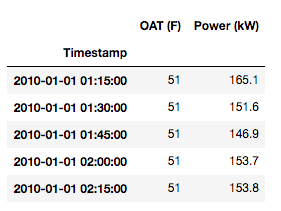Import the csv file into a dataframe.Make the first column as its index.Converts the index’s type from “object” to “datetime64[ns]” (this is a common gotcha!)

## Exploring the Dataset

Let’s first visualize the data by plotting it with pandas.

`df.plot(figsize=(18,5))`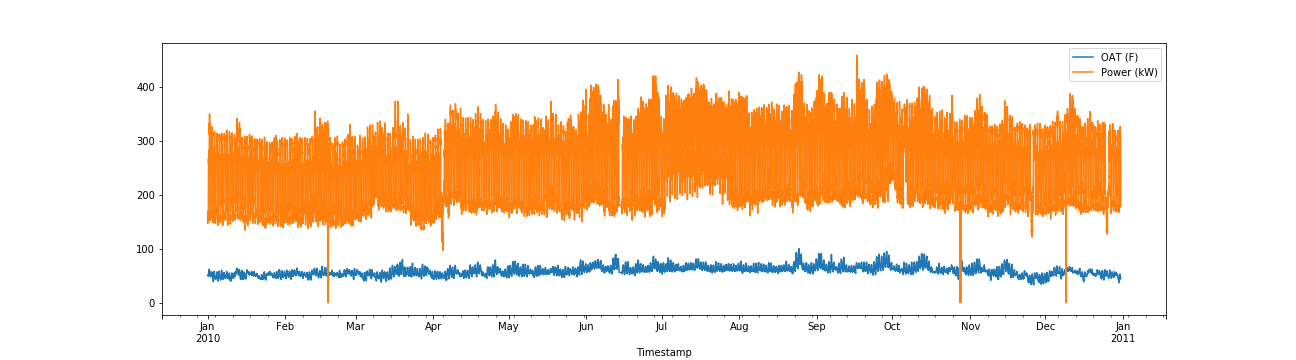Sweet! The x-axis shows that we have data from Jan 2010 — Dec 2010.

Bonus: Try plotting the data without converting the index type from object to datetime. Do you see any difference in the x-axis?

Upon closer inspection, you should notice two odd things about the plot,

There seems to be no missing data (very strange)There appear to be some anomalies in the data (long downward spikes)

Let’s tackle it one at a time.

## No missing data

It is nothing short of a miracle to work on a dataset with no missing values. This is why it’s imperative that we double check for null (missing) values before moving forward.

`df.isnull().values.any()`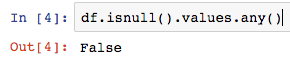The “False” output confirms that there are no null values in the dataframe.

Note: Since this is a publicly available dataset, it is likely pre-cleaned. However, when dealing with raw data, you can be certain to find missing values, hence the verification.

## Long spikes

The anomalies in the data are called “outliers” in the statistics world. Outliers are mostly (not always) the result of an experimental error (malfunctioning of the meter could be a probable cause) or it could be the correct value. Either way, it’s better to discard it.

Note: If you’ve thoroughly studied your regression concepts, you know that outliers can significantly affect the slope of the regression line; this is why it’s essential to remove them. If you want to dig further, here’s an article that describes the problem succinctly.

If the data follows a normal distribution, we can use the 68–95–99.7 rule to remove the outliers.

But first, let’s double check our assumption (remember — always be suspicious of the data and never make any assumptions) by running the following code,

`df.hist()`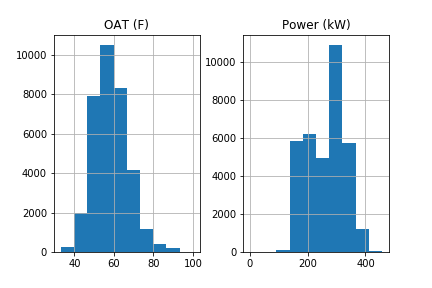.hist() creates one histogram per column, thereby giving a graphical representation of the distribution of the data. The graphs show that the data roughly follows a normal distribution.

Now let’s drop all values that are greater than 3 standard deviations from the mean and plot the new dataframe.

`std_dev = 3`
`df = df[(np.abs(stats.zscore(df)) < float(std_dev)).all(axis=1)]`
`df.plot(figsize=(18,5))`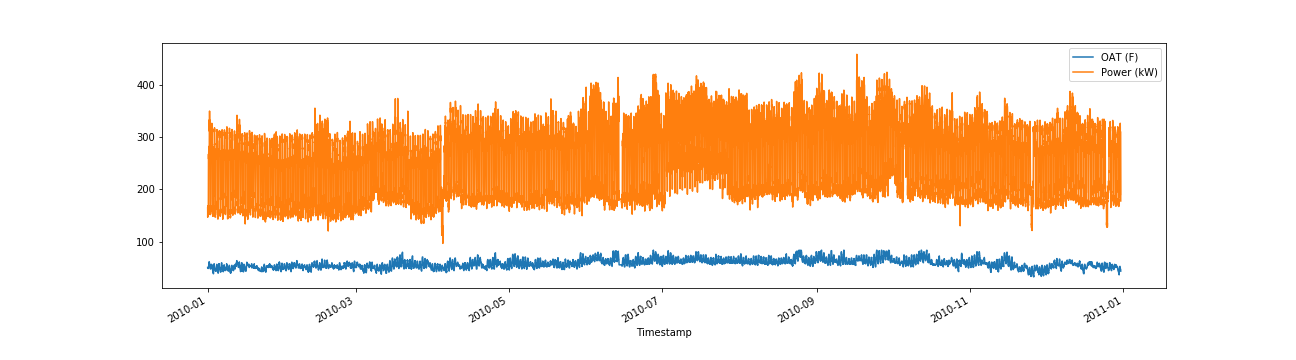Great! We have removed the spikes and successfully cleaned our data.

## Validate linear relationship

The title of this post makes it clear that OAT and Power have a linear relationship. But how do you find it out for yourself? A simple scatter plot should be enough,

`plt.scatter(df[‘OAT (F)’], df[‘Power (kW)’])`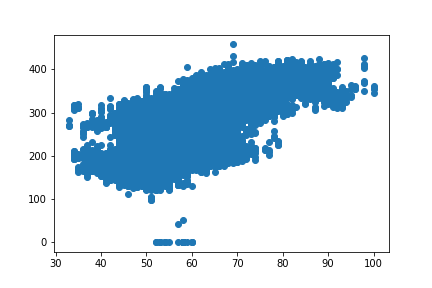## Check timezone

Last but not least, we need to verify the timezone of our dataset. Let’s pick a random day, say — 4th Mar 2010 (Thursday) and plot OAT.

`df.loc[‘2010–03–04’, [‘OAT (F)’]].plot()`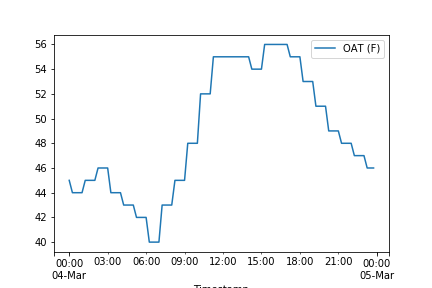OAT starts rising after sunrise (~6:30 am) and falls after sunset (5:30 pm) — which makes total sense. Therefore, we can infer that the data contains local timezone, i.e. PST since the building is in Fremont, CA, USA.

Lastly, let’s plot the Power of the building on the same day.

`df.loc[‘2010–03–04’, [‘Power (kW)’]].plot()`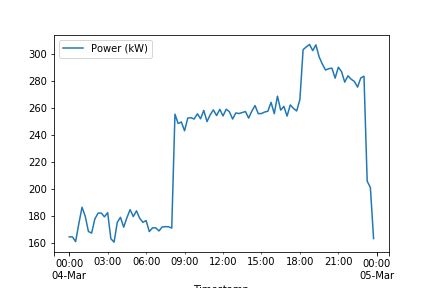You can see an increase in Power during 9am-11:30pm (probably the store’s opening hours?)

## Linear Regression

The moment you’ve all been waiting for! Scikit-Learn makes it extremely easy to run models & assess its performance. We will use k-folds cross-validation (k=3) to assess the performance of our model.

```X = pd.DataFrame(df[‘OAT (F)’])
y = pd.DataFrame(df[‘Power (kW)’])```
```model = LinearRegression()
scores = []
kfold = KFold(n_splits=3, shuffle=True, random_state=42)
for i, (train, test) in enumerate(kfold.split(X, y)):
model.fit(X.iloc[train,:], y.iloc[train,:])
score = model.score(X.iloc[test,:], y.iloc[test,:])
scores.append(score)```
`print(scores)`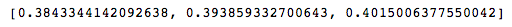Code Explanation: model = LinearRegression() creates a linear regression model and the for loop divides the dataset into three folds (by shuffling its indices). Inside the loop, we fit the data and then assess its performance by appending its score to a list (scikit-learn returns the R² score which is simply the coefficient of determination).

Hmm…that’s a bummer. These scores certainly do not look good.

How can we improve the model? Note that when we plotted the data for 4th Mar, 2010 the Power and OAT increased only during certain hours!

Bonus: Try plotting other random days, like a weekday vs a weekend and a day in June vs a day in October (Summer vs Winter) and see if you observe any differences.

## Time of Day

The target variable (Power) is highly dependent on the time of day. We will use this information to incorporate it into our regression model.

The following code does this by making use of one-hot encoding.

`X[‘tod’] = X.index.hour`
```# drop_first = True removes multi-collinearity
```# Add all the columns to the model data
```# Drop the original column that was expanded
X.drop(columns=[‘tod’], inplace=True)```
`print(X.head())`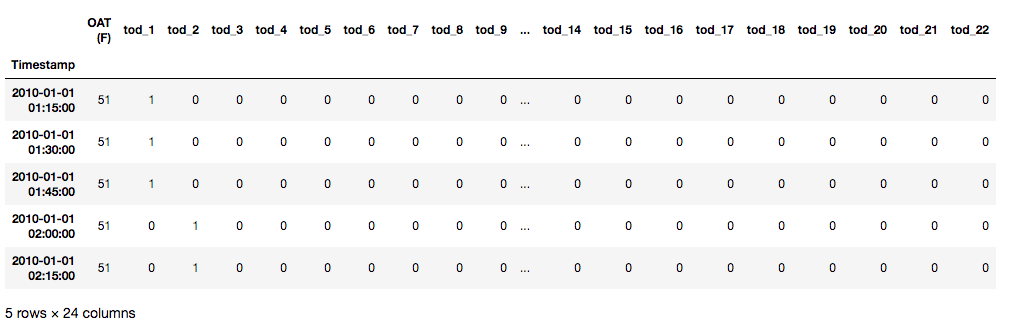Now let’s use k-folds cross-validation to assess the performance of our model again.

Note: We’re now dealing with multiple regression because there is more than one independent variable.
```model = LinearRegression()
scores = []
kfold = KFold(n_splits=3, shuffle=True, random_state=42)
for i, (train, test) in enumerate(kfold.split(X, y)):
model.fit(X.iloc[train,:], y.iloc[train,:])
scores.append(model.score(X.iloc[test,:], y.iloc[test,:]))```
`print(scores)`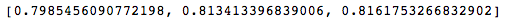There you go! What a big difference this made to our model!

### Conclusion

In this post, we learned the basics of exploring a dataset and preparing it to fit to a regression model. We assessed its performance, detected its shortcomings and fixed it by adding the time of day as a feature.

For further practice, I would encourage you to explore the other 8 buildings and see how day of week, day of year, and month of year compare against time of day. It’s as simple as changing X.index.hour to X.index.dayofweek, X.index.month… Refer pandas’ timestamp documentation.

That’s all folks!